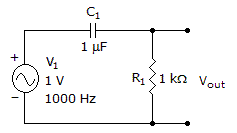# Electronics - Capacitors

### Exercise :: Capacitors - True or False

16.

The unit of capacitance is a farad; one farad stores one coulomb of charge or 6.24 x 1018 electrons.

 A. True B. False

Explanation:

No answer description available for this question. Let us discuss.

17.

The opposition to ac from a capacitor is called reluctance.

 A. True B. False

Explanation:

No answer description available for this question. Let us discuss.

18.

The greater the distance between the plates of a capacitor, the smaller the capacitance value.

 A. True B. False

Explanation:

No answer description available for this question. Let us discuss.

19.

An ohmmeter can be used to test a capacitor.

 A. True B. False

Explanation:

No answer description available for this question. Let us discuss.

20.

This circuit is an RC integrator.A. True B. False